Function Repository Resource:

# GoldbergGraph

Create a graph corresponding to a Goldberg polyhedron

Contributed by: Jan Mangaldan
 ResourceFunction["GoldbergGraph"][base,n] gives a graph corresponding to an order-n Goldberg polyhedron based on the polyhedron base. ResourceFunction["GoldbergGraph"][base,class,n] gives a graph corresponding to an order-n Goldberg polyhedron of class class, based on the polyhedron base.

## Details and Options

Possible values of base are "Tetrahedron", "Octahedron" or "Icosahedron".
Possible values of class include "I", "II", 1 and 2.
ResourceFunction["GoldbergGraph"][base,n] is equivalent to ResourceFunction["GoldbergGraph"][base,2,n].
ResourceFunction["GoldbergGraph"][base,1,n] or ResourceFunction["GoldbergGraph"][base,"I",n] generates a three-dimensional graph corresponding to the class I (n+1,0) Goldberg polyhedron, the dual polyhedron of a geodesic sphere.
ResourceFunction["GoldbergGraph"][base,2,n] or ResourceFunction["GoldbergGraph"][base,"II",n] generates a three-dimensional graph corresponding to the class II (n,n) Goldberg polyhedron.
ResourceFunction["GoldbergGraph"] takes the same options as Graph3D.
With the setting VertexCoordinates"Embedded", coordinates corresponding to the vertices of the Goldberg graph are generated with a special method.

## Examples

### Basic Examples (2)

Generate a Goldberg graph corresponding to the truncated icosahedron (buckyball):

 In:=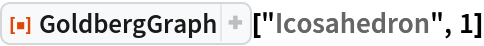Out=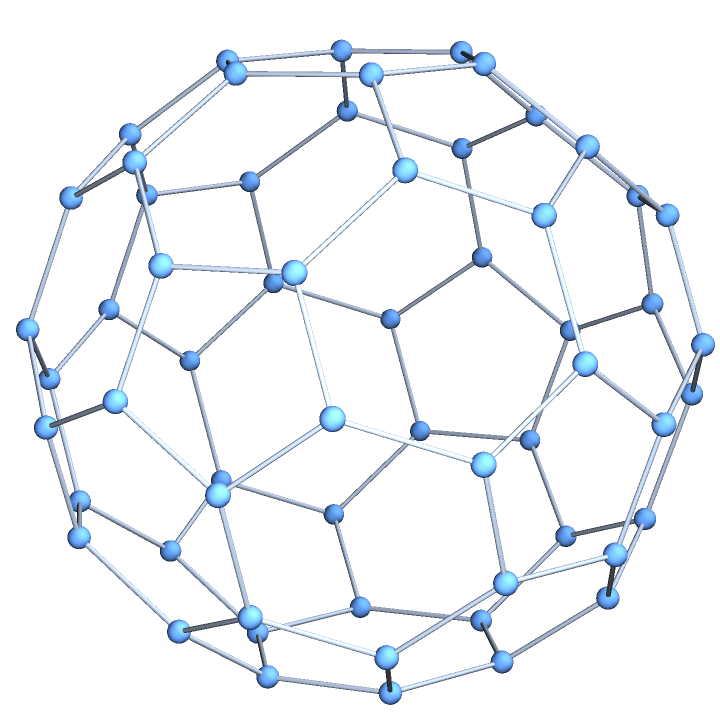The result is isomorphic with a truncated icosahedron:

 In:=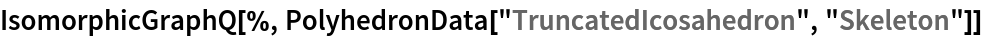Out=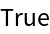Generate a class I, order-3 Goldberg graph with an octahedral base and specially computed coordinates for the vertices:

 In:=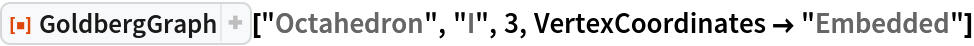Out=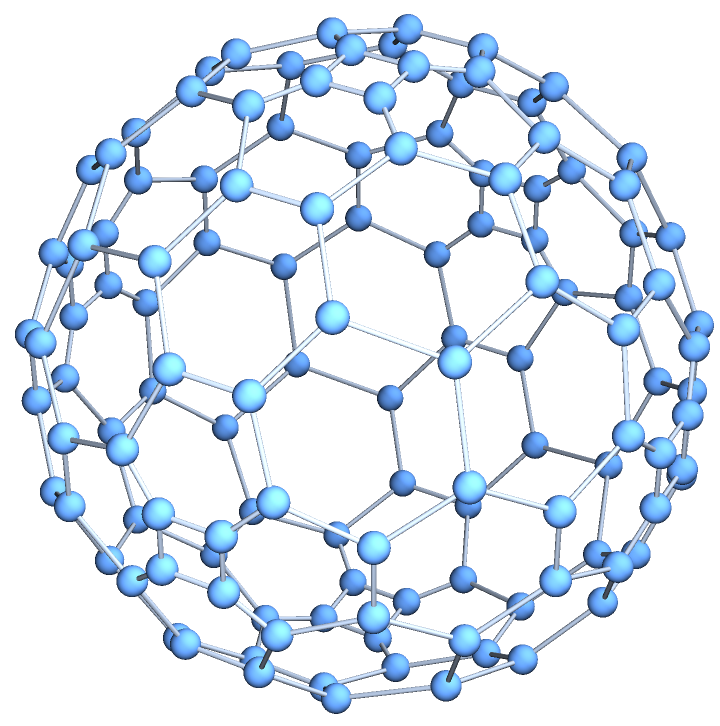### Scope (2)

Goldberg graphs corresponding to the Platonic solids:

 In:=Out=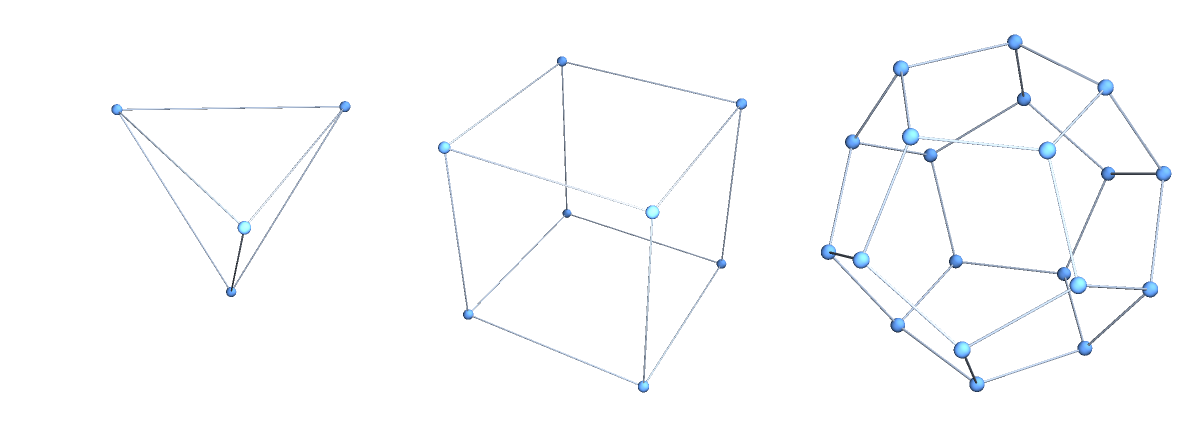Generate a class I Goldberg graph with a tetrahedral base:

 In:=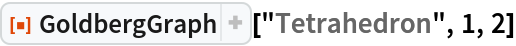Out=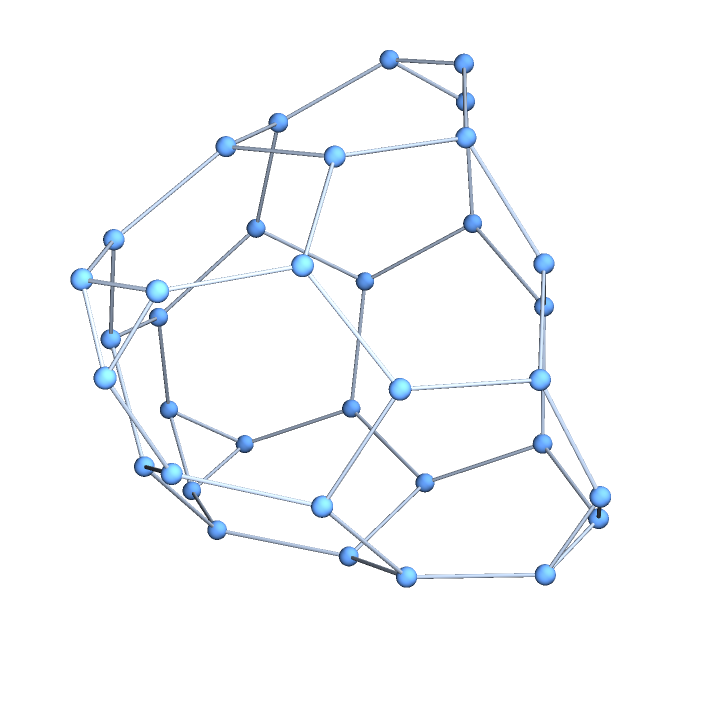Convert to a standard Graph object:

 In:=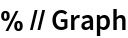Out=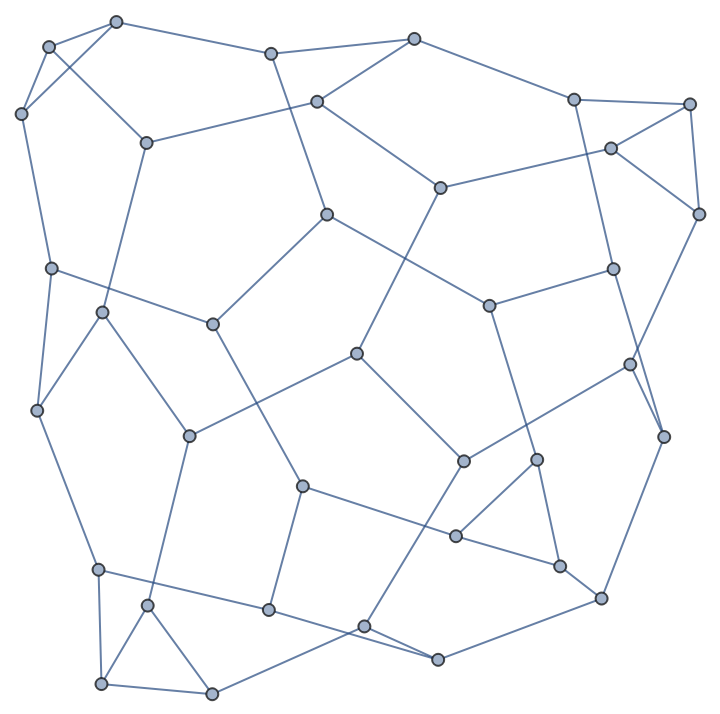### Options (4)

#### GraphLayout (1)

Specify various layouts for the Goldberg graph:

 In:=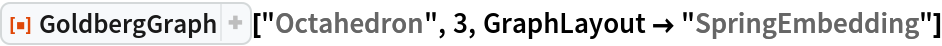Out=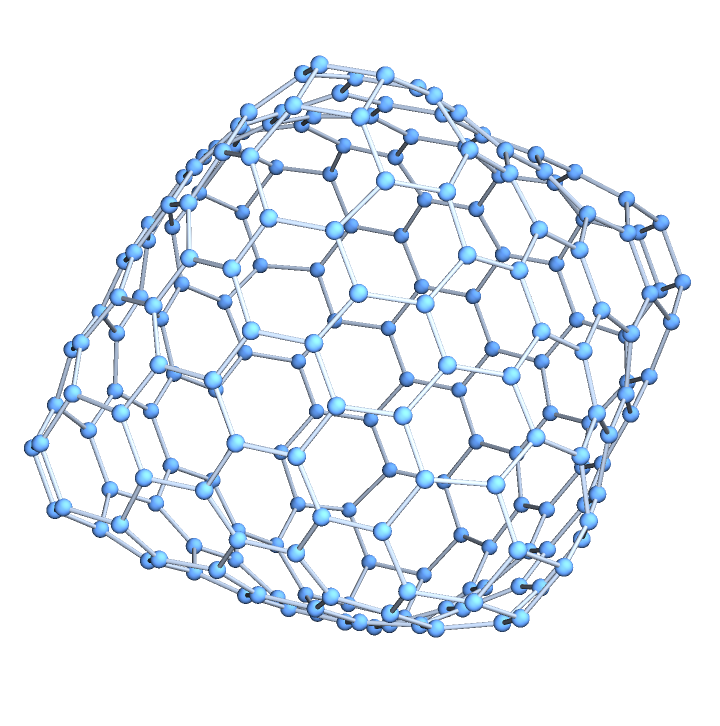In:=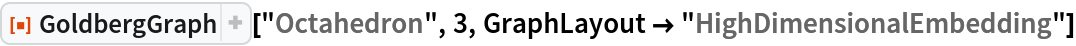Out=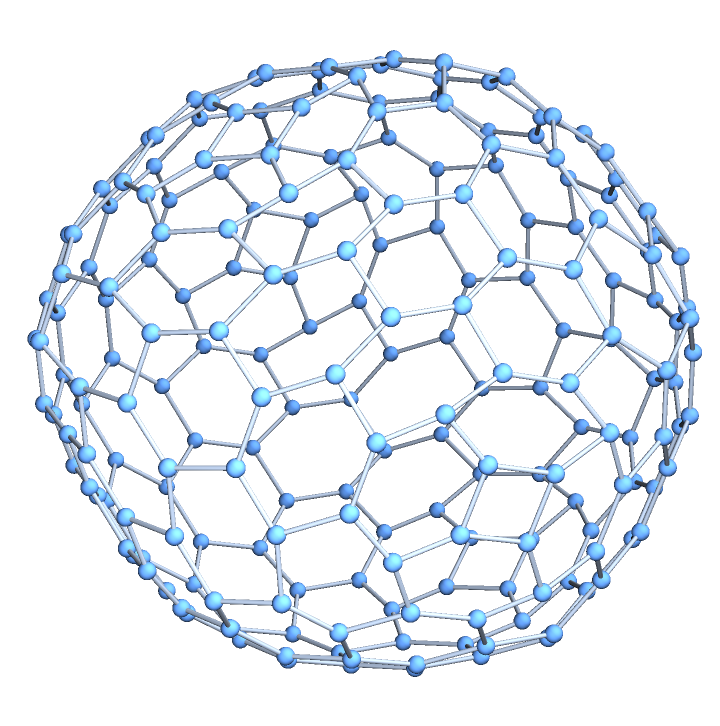#### PlotTheme (1)

Use a large graph theme:

 In:=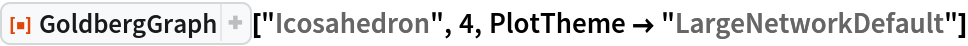Out=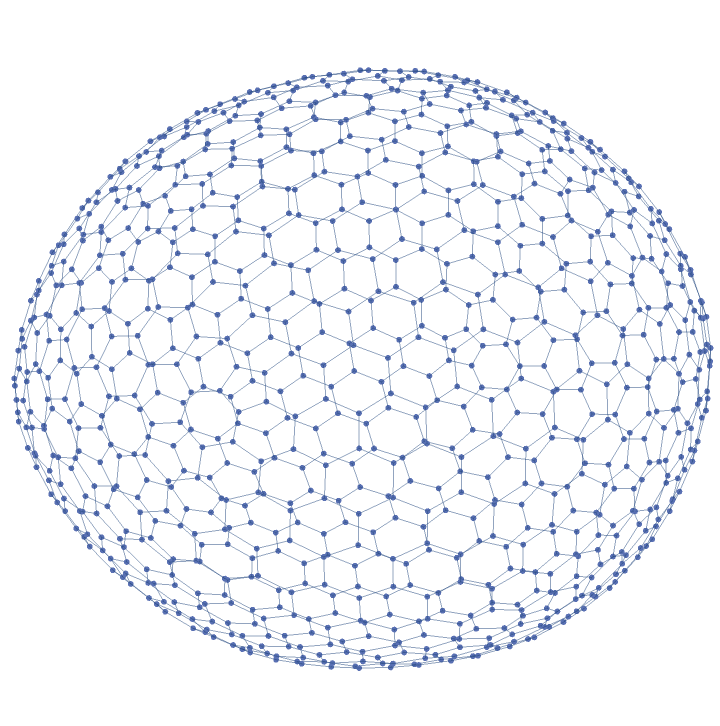#### VertexCoordinates (2)

By default, vertex coordinates are computed automatically, depending on the setting for GraphLayout:

 In:=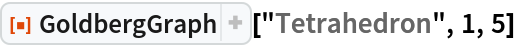Out=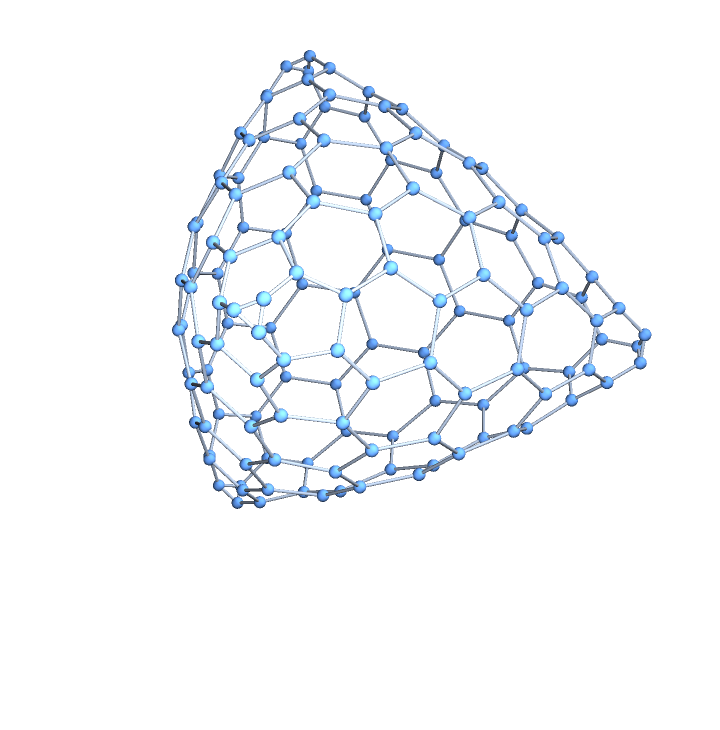Use specially computed coordinates for the vertices:

 In:=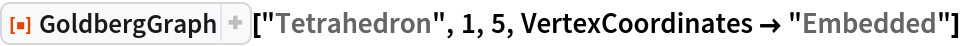Out=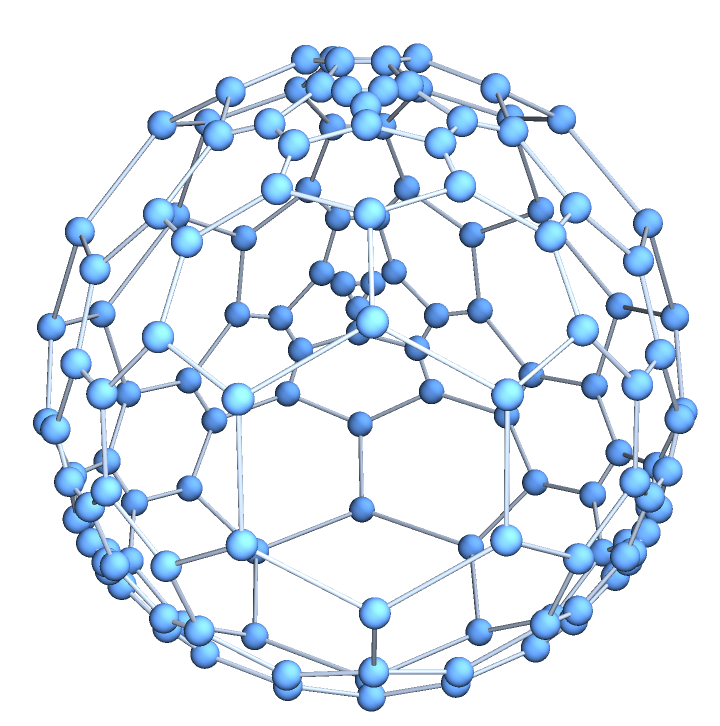### Properties and Relations (1)

Resource function BuckyballGraph is a special case of GoldbergGraph:

 In:=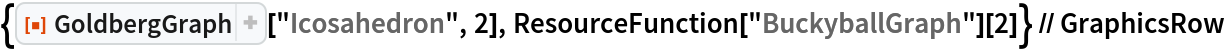Out=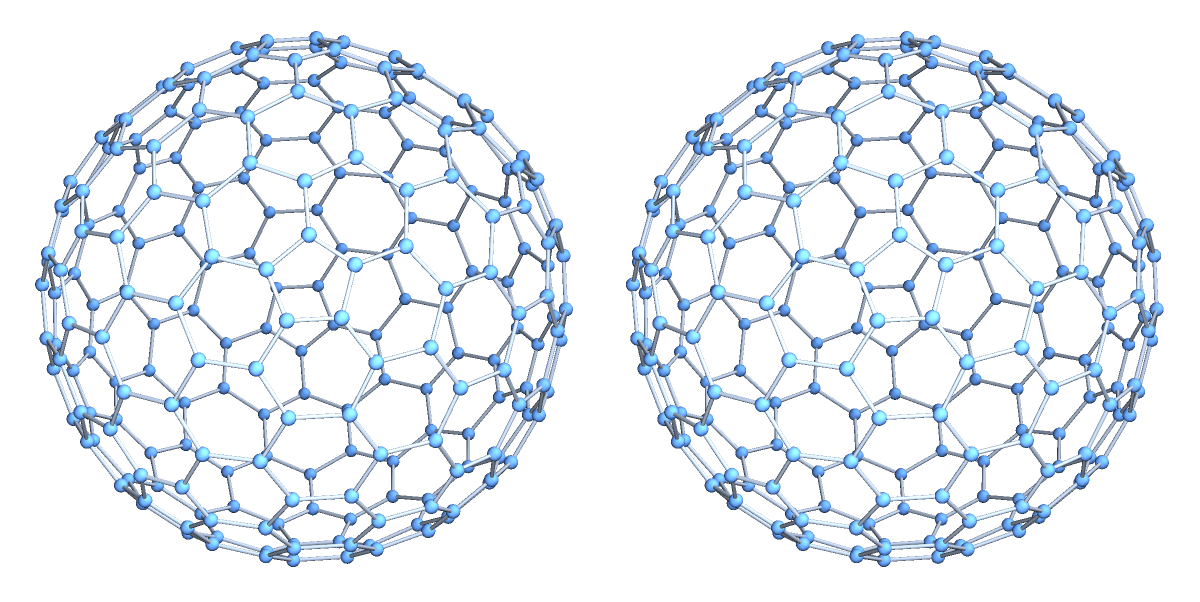## Version History

• 1.0.1 – 24 January 2022
• 1.0.0 – 10 February 2021